## Indicadores

•Citado por SciELO
•Acessos

## Links relacionados

•Citado por Google
•Similares em SciELO
•Similares em Google

## versão On-line ISSN 1657-4214

### Perf. de Coyunt. Econ.  no.19 Medellín jun. 2012

ARTICLES

Estimating Portfolio Value at Risk with GARCH and MGARCH models*

Estimación de valor de la cartera en riesgo con los modelos GARCH y MGARCH

María Isabel Restrepo E.**

**Docente de Economía, Universidad de Antioquia. Dirección electrónica: mirestrepo@economicas.udea.edu.co.

-Introduction. –I. Theoretical Framework. II. Estimation and Results. –III. Conclusions -

Primera versión recibida: Marzo 27 de 2012; versión final aceptada: Julio 17 de 2012

ABSTRACT

The aim of this paper is to estimate GARCH models, univariate and multivariate, for the daily returns of a portfolio consisting of five Colombian financial market assets, in order to evaluate which shows better performance in estimating the Value at Risk of the portfolio. To calculate VaR, with a confidence level of 95%, equal weight is assigned to the assets in the portfolio. The results show that the univariate GARCH models outperform the MGARCH in estimating the VaR of the portfolio.

Key words: GARCH models, MGARCH models, Value at Risk.

RESUMEN

El objetivo de este artículo es estimar algunos modelos GARCH, univariados y multivariados, para los retornos diarios de un portafolio compuesto por cinco activos del mercado financiero colombiano, con el fin de evaluar cual muestra mejor desempeño en el cálculo del Valor en Riesgo del portafolio. Para calcular el VaR, con un nivel de confianza del 95%, se le asigna igual peso a los activos en el portafolio. Los resultados muestran que los modelos GARCH univariados tienen mejor desempeño que los MGARCH en la estimación del VaR del portafolio.

Palabras claves: Modelos GARCH, Modelos MGARCH, Valor en Riesgo.

JEL: C22, G11.

Introduction

Due to the increased volatility of financial markets in recent decades, the modeling of risk and volatility of financial instruments using various statistical tools has become a major area of research. In particular, major emphasis has been placed on modeling the temporal dependencies present in conditional higher-order moments, in order to achieve a more efficient management of risk associated with fluctuations in these variables.

Since the pioneering work of Engle (1982) who develops an Autoregressive Conditional Heteroskedasticity model (ARCH), there have been produced major advances in modeling and forecasting the volatility of a time series. It has been shown that the family of ARCH models captures much empirical regularity associated with the volatility of returns on financial assets such as leptokurtic distributions, volatility clustering, leverage effects, persistence and asymmetric volatility, among others (Bollerslev et al., 1994; Ghysels et al., 1996; Engle et al., 2001).

The heteroskedastic nature of the financial assets returns implies that the ARCH methodology is a natural candidate for its modeling. However, many works in this area are made in the multivariate context, because the volatility of financial markets moves over time and across different assets and markets. In addition, multivariate models allow estimating efficiently the dynamic cross-correlations that may exist between the returns of a set of assets, which is a crucial factor in determining the gains from portfolio diversification (Bera and Kim, 2002).

One of the most common measures to assess the risk of a portfolio is the Value at Risk. The VaRαcorresponds to the αth quantile of the distribution of profits and losses of a portfolio. That is, represents the maximum loss incurred by an asset in the best α * 100 % best cases (smaller losses).

This paper seeks, with information on daily returns of five assets of the Colombian market from 01/03/2008 until 10/12/2010, to estimate a univariate and a multivariate GARCH model for a portfolio, with information on daily returns of five assets of the Colombian stock market. Assigning equal weights for the asset returns, I use the VaR as a portfolio risk measure, in order to make comparisons between the two models.

I. Theoretical Framework

Value at Risk –VaR

The importance of this measure lies in its usefulness for quantification of market risk and as a regulatory tool, since the environment in which financial activity unfolds is characterized by large fluctuations in prices of financial assets. In general, the VaR is an indicator of the extreme points within which fluctuates the profitability of an investment in an asset or a particular portfolio. That is, represents the maximum loss incurred by an asset in theα * 100% best cases (smaller losses). It is expected that the loss on the investment does not exceed the VaR with probability α.

On the other hand, VaR is a natural application of volatility models given that it depends directly to the forecast of the conditional variance, consequently, the estimation of the conditional distribution of returns becomes the main input for the application of this methodology (Gallón and Gómez, 2007). Using the conditional mean and the conditional variance obtained from the estimated GARCH, the quantiles of the conditional distribution can be easily obtained for the calculation of VaR (Tsay, 2002). According to the distribution of errors, the VaR can be calculated as: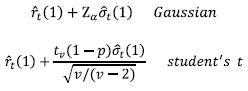Where Zα is the αth quantile of a standard normal distribution and tv(1 - p), is the (1 - p)th quantile of a student's t distribution with v degrees of freedom.

As mentioned by Tsay (2002), VaR is associated with the prediction of a possible loss of a portfolio for a given time horizon. Therefore, it should be calculated using the distribution of forecasts of returns. Specifically, the VaR of the returns of a portfolio, rt, for a horizon s should be estimated based on forecasts of rt+s(s) given the information available at time t. The forecast of rt+s(s) depends on the model assumed to describe the dynamics of returns, such as the ARCH model.

According with Alves, Nogales and Ruiz (2009) the first decision one has to make when trying to predict the VaR of a portfolio is whether to use a multivariate model for the system of individual asset returns or, alternatively, to use a univariate procedure for the portfolio returns. They argue that, as the dimension of the portfolio increases, the usually large number of parameters involved renders the estimation of multivariate models more complicated, compromising their predictive ability.

Univariate GARCH models

First, it is important to note some stylized facts of financial assets (in high frequency). The distribution of returns is not normal: extreme returns occur most frequently than expected under normality (fat tails) and extreme negative returns occur most frequently than positive (negative asymmetry); autocorrelations of the returns tend to be not significant, there are clusters of volatility and calendar effects (Melo et al., 2005). According to (Tsay, 2010), a basic way to represent this type of series would be: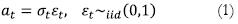Where αt is the shock or innovation of the asset returns, rt, and σt2 is the process for the volatility of rt. Conditional heteroskedasticity models center in the the dynamics of σt2: The volatility equation. In order to do that, the specification in equation (1) is the starting point. It can be expressed as: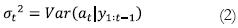Based on the type of specification for equation (2), we can separate conditional heteroskedastic models into (i) deterministic models ''that use an exact function to govern the evolution of σt2'' (Tsay, 2010, p. 100), or (ii) stochastic models, that make σt2 follow a random process. (G)ARCH and E-GARCH models, for example, are located in the first group, while stochastic volatility models, belong to the second group.

GARCH (generalized autoregressive conditional heteroskedastic) models have a more flexible lags structure, and in many cases, allow a description more parsimonious of the data than ARCH models. In this case, we keep the basic expression for innovations, and use the following expression for the volatility equation:Where the persistence of shocks to the volatility is given by the sum of αi and βi. This expression is useful in representing the clustering in volatility usually observed in series of returns: a large shock in volatility yesterday increases the probability of a large shock in today's volatility (Tsay, 2010). However, a drawback of this model is that it does not allow for distinguishing between impacts of negative and positive shocks, which tend to be different (leverage effect). Some regularity conditions must be imposed to the model: ω > 0, αi > 0, βf > 0,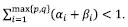.

The positivity restrictions are necessary for the conditional variance of the innovations to exist, and for avoiding degeneration of their process αt (Carnero, Peña, & Ruiz, 2004). The last constraint implies that the process is covariance stationary, and that its marginal variance of innovations exists (σ2 < ∞), but its conditional variance, σt2, evolves over time [ (Tsay, 2010) (Carnero, Peña, & Ruiz, 2004)].

On the other hand, the EGARCH model relaxes the positivity restrictions to its parameters and allows for the treatment of leverage effects. In that sense, the EGARCH considers the following specification for the weighted innovation: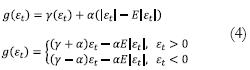Note that the first term, related to is a regular ARCH term and it determines the sign of the effect, while the second one, linked to α is the asymmetric effect and it determines the size of the effect. This specification succeeds at representing leverage effects, since usually the estimated value of γ is negative, which implies that |γ - α|>|γ + α|. Now, in order to lose the positivity constraints, the volatility equation is expressed in log terms. For instance, in the case of EGARCH(1,1), it is given by: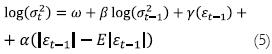Regarding restrictions, the only restriction imposed on the parameters of the EGARCH is that |β| < 1, so that the log-volatility process is stationary.

Finally, as mentioned above, given an estimated uniariate (G)ARCH model on a return series, one knows the conditional distribution of returns, and one can forecast the value-at-risk (VaR) of a long or short position. When considering a portfolio of assets, the portfolio return can be computed directly from the asset shares and returns (Bauwens et al., 2006).

Multivariate GARCH models

A multivariate GARCH(p,q) model, MGARCH(p,q), can be represented as: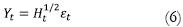Where Ht1/2 is the conditional covariance matrix of Yt (series of returns) and εt is a vector of white noise processes such that E(εt) = 0 and E(εtε't) = 1. There are two kinds of multivariate GARCH models: one type models the time-varying covariances and the second type models the conditional correlations (Tsay, 2010).

The most direct generalization of the univariate GARCH(1,1) model is the VEC model given by: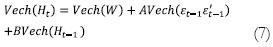where the vec operator allows each cross-product to influence each covariance term. The VEC model is covariance stationary if the modulus of the eigenvalues of A+B are less than one.

One of the main drawbacks of the multivariate models, and from this in particular, is that the number of parameters to be estimated is too large. Therefore, the DVEC model restricts the matrices A and B to be diagonal. In this case, each element hijt depends only on its own lag and on the previous value εitεjt. However, this model is not suitable to represent volatility transmissions between assets and in both VEC and DVEC models it is difficult to guarantee the positiveness of Ht (Tsay, 2010).

Another usual specification is the BEKK model based on quadratic forms: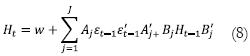The full BEKK becomes intractable as the number of assets grows. However, the diagonal BEKK partially addresses some of the number of parameters by restricting A and B to be diagonal.

Finally, the last multivariate model that is going to be considered here is the Constant Conditional Correlation (CCC), which uses a different approach: it decomposes the conditional covariance into the conditional variances and the conditional correlation, which is assumed to be constant: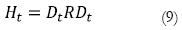Where is a diagonal matrix with the conditional standard deviation of the ith asset on its diagonal position. In this model, the conditional variances are typically model using standard GARCH(1,1) models: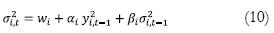II. Estimation and Results

Data

In this paper I consider some of the five most tradable assets in the stock exchange in Colombia. The returns of these assets were calculated from the logarithmic difference of the price. These returns are for Éxito, Bancolombia, Nutresa, Isa and Ecopetrol. Additionally I consider the IGBC1 –Índice General de la Bolsa de Valores de Colombia–, to compare it with the results obtained by forming a portfolio with equal weights for those returns. The data has daily frequency from 03/01/2008 until 10/12/2012 for a total of 758 data. Below are the plots of the returns and the descriptive statistics.

In all cases I apply stationarity tests (Dickey-Fuller and Phillips-Perron) and reject the unit root null hypothesis. Below are the QQ plots of returns. It compares the observed quantiles with the theoretical quantiles of a normal distribution. Therefore, if the distribution of the series is normal it should be a straight line.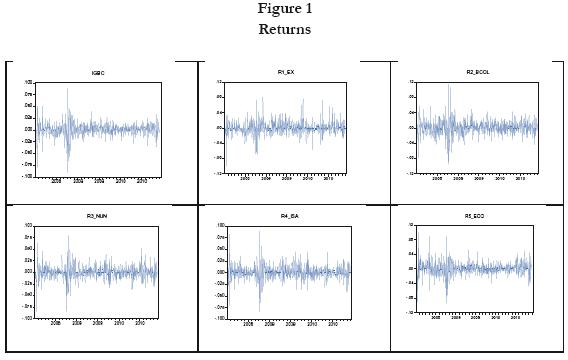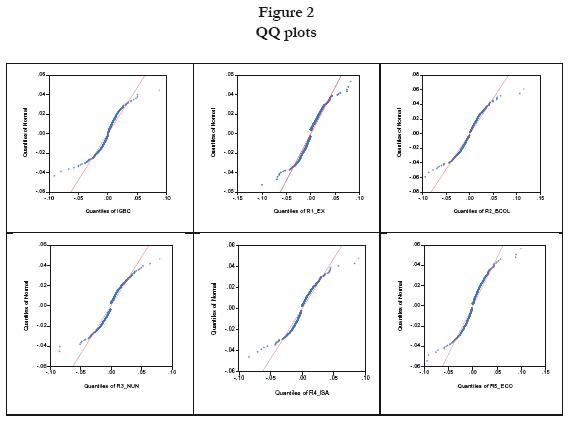In this Figure, there is a pattern of ''S rotated'' in all cases, where the greatest differences with respect to dotted line are at the extremes. This result indicates that the distributions of the series have tails heavier than those of a normal distribution. Below there is a summary of statistics for each return: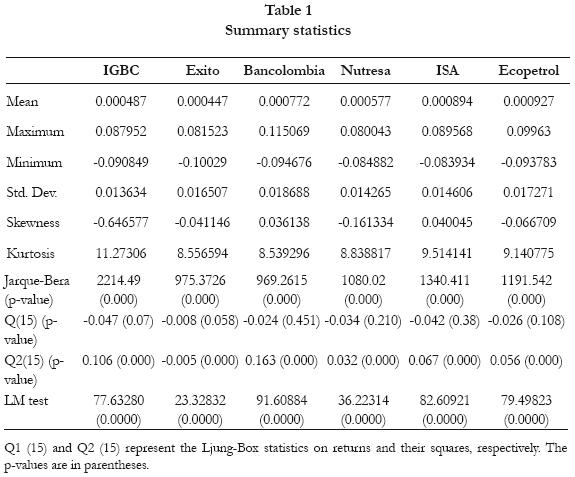Considering the standard deviation as a measure of volatility, more volatile returns correspond to, in order, Bancolombia, Ecopetrol and Exito. On the other hand, according to the coefficients of skewness and kurtosis, the Jarque-Bera statistic and QQ plots, one cannot assume normality of returns. Additionally, according to the Ljung-Box statistics we cannot reject the null hypothesis of no autocorrelation up to order 15 in the series of returns but is rejected in the squared returns. Finally, to confirm whether there are ARCH effects, was applied the Lagrange multiplier test (LM test), which considers the null hypothesis of no ARCH errors versus the alternative hypothesis that the conditional error variance is given by an ARCH process. The null hypothesis is rejected in all cases, confirming ARCH effects in the errors.

In the estimation, first, I consider two univariate models, one for the IGBC and one for the portfolio assuming equal weights for assets. In both cases I tested various specifications and the model with best fit given the constraints of the parameters, the significance of the conditional volatility and the AIC and SC criteria was an EGARCH (1,1) with student's errors.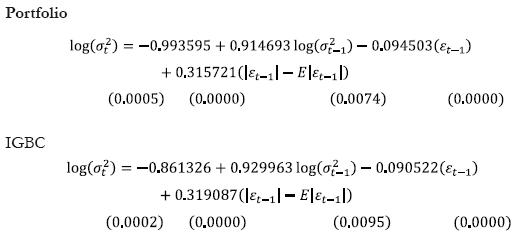The fact that both series have similar behavior has sense because the portfolio was constructed from some of the more tradable assets in the Colombian market and IGBC is an indicator that measures just that. In both cases it holds that Β < 1 (significant) and is verified that there are leverage effects due to the parameter γ is negative, so the effect on volatility is bigger when the return is negative than when it is positive. Below are the QQ plots of the standardized residuals. It is observed that normality for the standardized residuals is still being rejected due to the excess of kurtosis but in a lower degree.

In order to check the specification in the conditional variance function in both models I used the LM test, finding evidence for the absence of additional ARCH effects, confirming that the EGARCH (1,1)2 fits well.

In the multivariate case, three specifications were chosen to model the distribution of conditional covariance matrix of the returns of the five assets, : DVEC, DBEKK and CCC models. The choice of these models is not only because in relative terms they are simpler to estimate, but also because the cross-correlation of returns3 does not confirm a significant linear relation both contemporary and in the first lag, so there seems not to exist an important cross-influence on the markets. Additionally, I used the LM test and I found evidence for the existence of multivariate ARCH effects (at 5% significance). Then, I proceed to estimate the MGARCH models and the results are presented bellow. In all cases, the stationarity conditions are verified.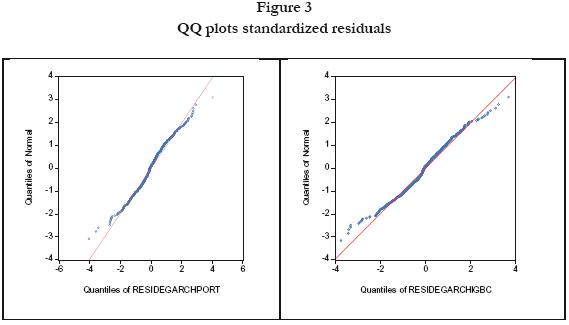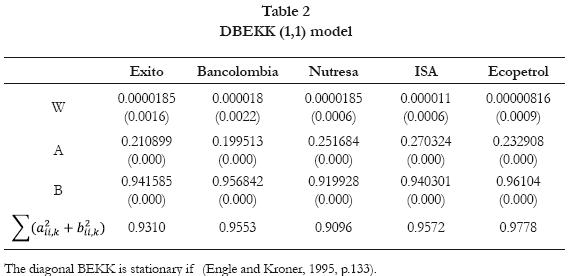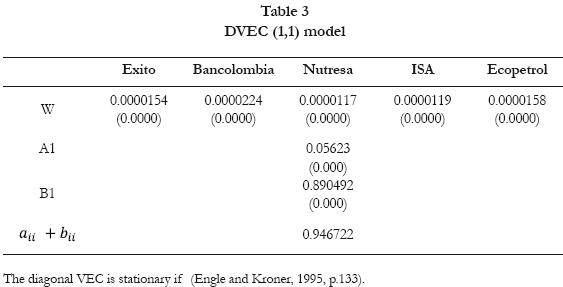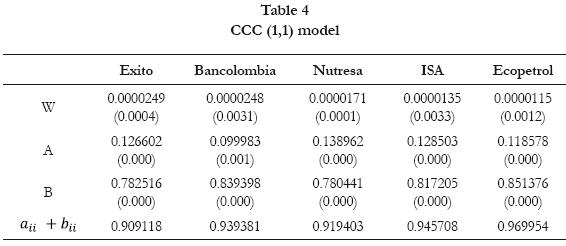The stationarity conditions are the same as in the univariate GARCH model W > 0, α, β > 0, aii + bii < 1. In the Appendix are the results for the estimated cross-correlation matrix from the CCC model.

The plots of the estimated conditional variances from the three models are presented below.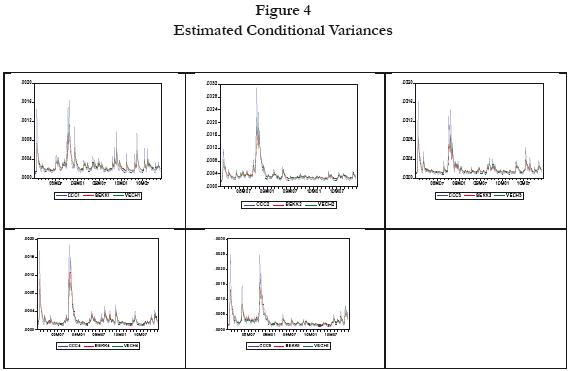In conclusion, it seems clear that the estimated conditional covariance matrices of the models are very close, however there is uncertainty about which of the models is better. In addition, QQ plots of standardized residuals are presented below, which are showing excess kurtosis in some cases with a result similar to the univariate models.

Once established the processes that model the dependence of returns, it is possible to calculate risk measures and then verify their performance through backtesting. Given the results presented above the VaR was estimated assuming that the distribution of the errors is a Student's , according to the methodology previously presented. In the multivariate case, following Gallón and Gómez (2007), the VaR for a portfolio consisting of N assets in a time horizon with probability is defined as: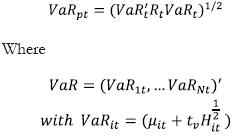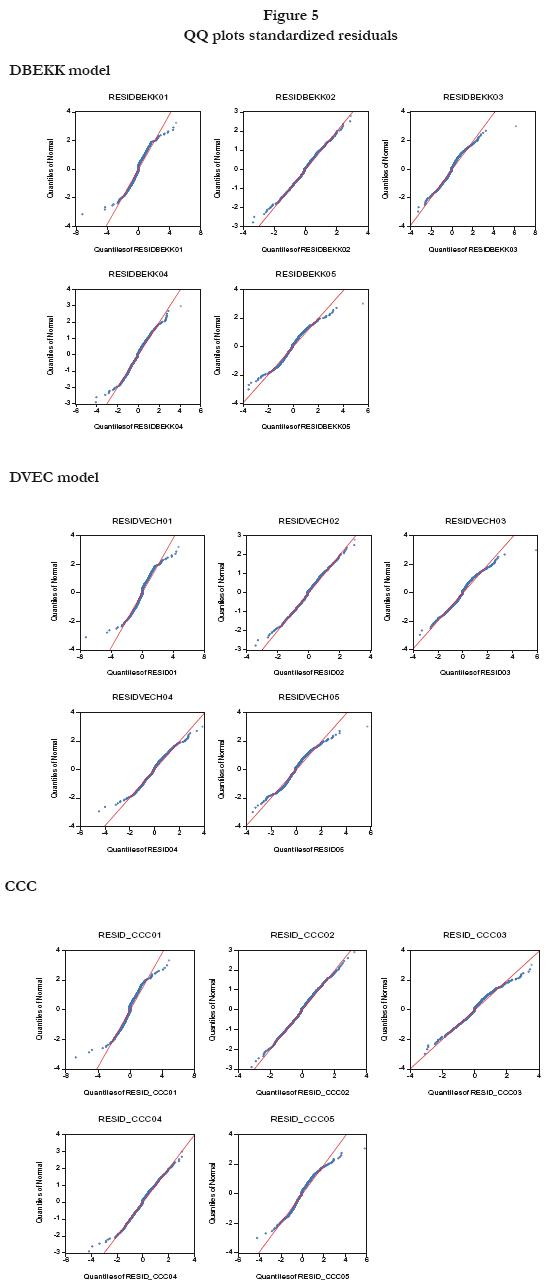Where wi is the amount invested in the ith asset. Here it is assumed that is wi equal for all assets, in order to compare it with the portfolio estimated in the univariate case and the IGBC.

A basic measure for comparing the estimated models is the failure ratio or HIT, defined as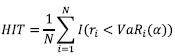Where l(ri < VaRi(α))is an indicator variable that takes the value 1 when ri < VaRi(α). A model is correctly specified when this ratio is equal to the pre-specified probability for the VaR. Below is the result of the estimate for a confidence level of 95%.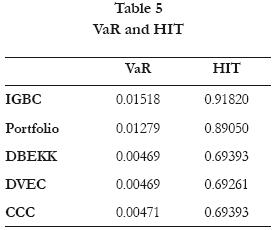For instance the VaR of the portfolio indicates that the maximum loss expected with a probability of 95% and a horizon of one day shall not exceed that percentage of the amount invested. The HIT calculated shows that the models that are closer to 95% pre-specified for the VaR are both models that were obtained from the univariate (E)GARCH. Additionally it is noted that similar results are obtained for the three multivariate specifications. Finally, the most important result is that the univariate models perform better in estimating the VaR than the multivariate models, which seems to underestimate the risk of the portfolio.

Conclusions

The modeling of risk and volatility of financial instruments has become a major area of research in last decades. This study sought to estimate the risk of a portfolio consisting of 5 assets of the Colombian stock market. In the estimation, I considered two univariate models, one for the IGBC and one for the portfolio assuming equal weights for assets. In the multivariate case, three specifications were chosen to model the distribution of conditional covariance matrix of the returns of the five assets: DVEC, DBEKK and CCC models. Once established the processes that model the dependence of returns, the VaR was estimated.

Results show how univariate models performed better in estimating the VaR than the multivariate models, which seems to underestimate the risk of the portfolio. However, the results are subject to the number of assets and the estimated multivariate models. In that sense, it would be interesting to consider more assets to make the estimation and use other multivariate GARCH specifications. Additionally, one could apply more sophisticated backtesting techniques and out of sample analysis. Finally, for comparisons would be interesting to consider more elaborated measures for the calculation of VaR such as the Conditional Autoregressive VaR (CaViaR).

NOTAS

* El artículo no tiene una investigación previa.

1 This is the result of weighting the more liquid shares with higher capitalization traded on the stock market.

2 For the IGBC the contrast yields a value of 1.759175 (0.1847) and for the portfolio 0.512384 (0.4741).

3 See Appendix

References

Alves, A.; Nogales, F.; Ruiz, E. (2009). ''Comparing univariate and multivariate models to forecast portfolio value-at-risk''. Statistics and Econometrics Working Papers ws097222, Universidad Carlos III, Departamento de Estadística y Econometría.         [ Links ]

Bauwens, L.; Laurent, S.; Rombouts, J. (2006). ''Multivariate GARCH models: A survey''. Journal of Applied Econometrics, 21, 79-109p.         [ Links ]

Bera, A. K.; Kim, S. (2002). ''Testing constancy of correlation and other specifications of the BGARCH model with an application to international equity returns''. Journal of Empirical Finance 9, 171–175.         [ Links ]

Bollerslev, T.; Engle, R.; Nelson, D. (1994). ''ARCH models''. En MacFadden, D. F., Engle, R. E. (Eds.), Handbook of Econometrics, Vol. 4. North-Holland: Amsterdam.         [ Links ]

Carnero, M. A.; Peña, D.; Ruiz, E. (2004). ''Persistence and Kurtosis in GARCH and Stochastic Volatility Models''. Journal of Financial Econometrics, 2 (2), 319-342.         [ Links ]

Engle, E. (1982). ''Autoregressive conditional heteroskedasticity with estimates of the variance of United Kingdom inflation''. Econometrica 50, 987–1008.         [ Links ]

Engle, R.; Kroner, K. (1995). ''Multivariate Simultaneous Generalized ARCH''. Econometric Theory Vol. 11, No. 1, pp. 122-150.         [ Links ]

Engle, R.; Patton, A. (2001). ''What good is a volatility model?'' Quantitative Finance 1, 237–245.         [ Links ]

Gallón, S.; Gómez, K. (2007). ''Distribución condicional de los retornos de la tasa de cambio colombiana: un ejercicio empírico a partir de modelos GARCH multivariados''. Revista de Economía del Rosario, Vol. 10, No. 2.         [ Links ]

Ghysels, E.; Harvey, A.; Renault, E. (1996). ''Stochastic Volatility''. En Maddala, G. S., Rao, C. R., Vinod, H. D., (Eds.), Handbook of Statistics, Vol. 14: statistical methods in finance. North-Holland: Amsterdam: 119–91.         [ Links ]

Melo, L.; Becerra, O. (2005). ''Medidas de riesgo, características y técnicas de medición: una aplicación del VaR y el ES a la tasa interbancaria de Colombia''. Borradores de Economía 343, Banco de la Republica de Colombia, 75 p.         [ Links ]

Tsay, R. (2002). ''Analysis of Financial Time Series.'' John Wiley & Sons.         [ Links ]

Tsay, R. (2010). Analysis of Financial Time Series. Chicago: John Wiley & Sons, Inc.         [ Links ]

Appendix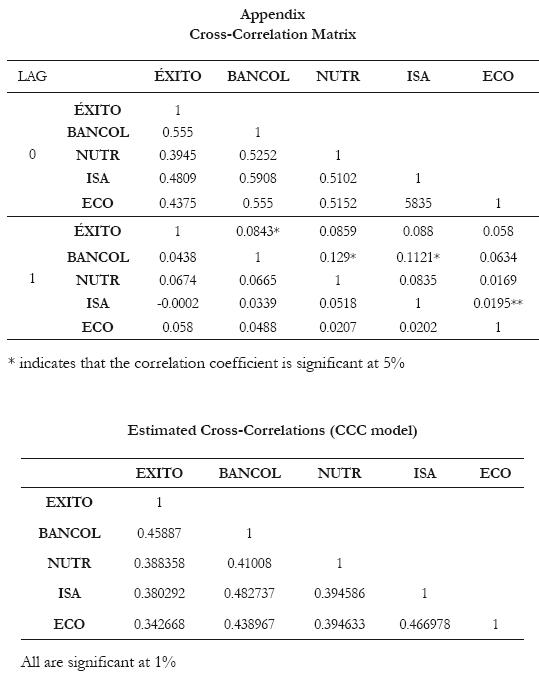Todo o conteúdo deste periódico, exceto onde está identificado, está licenciado sob uma Licença Creative Commons